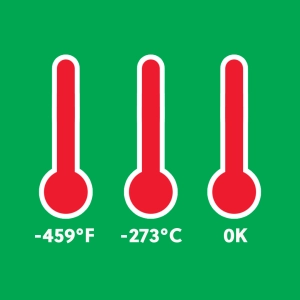#Fahrenheit Celsius Kelvin

Temperature is a fundamental physical quantity

Illustration of the three commonly used systems to measure temperature: Fahrenheit, Celsius and Kelvin.

The Celsius scale, the one most widely used, is centigrade: 100 divisions separate the freezing and boiling points of water. In what is now referred to as the English (or American) system, water’s freezing and boiling points are separated by 180°. The Kelvin scale (or Absolute scale) is primarily used only in Science.

• The formula to convert Celsius to Fahrenheit is F = 9*C/5 + 32
• The formula to convert Fahrenheit to Celsius is C = 5*(F - 32)/9
• The formula to convert Celsius to Kelvin is simply  K = C + 273

Click and drag the temperature indicated by each thermometer.